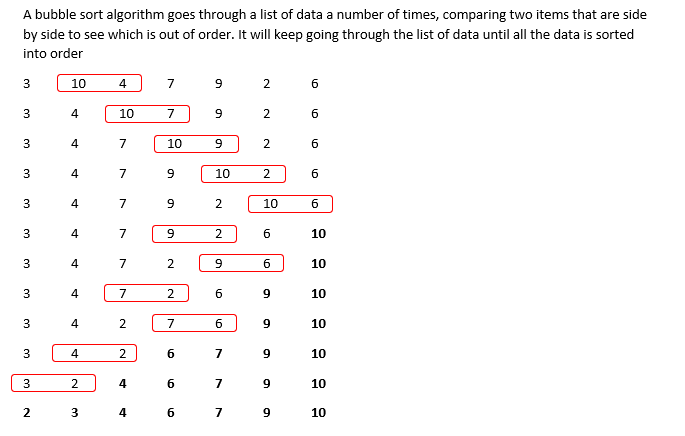# Capgemini Technical MCQ's Previous Year Questions

28 January 2023

Capgemini Technical MCQ's Previous Year Questions

Check below videos for previous year questions:

Pseudocode Sample Questions:

Game based Aptitude Questions:

Capgemini Technical MCQ's Previous Year Questions

Q1)  Which of the following statements is incorrect for Linked list data structure?

A. Memory allocation from Heap

B. Memory allocation from stack

C. Size is not fixed.

D. It occupies more memory than array.

Explanation:

LinkedList is not stored in a contiguous memory location. Each element int the list is spread across the memory and are linked by the pointers in the Node. Thus, whenever a new element needs to be added a separate memory is allocated enough to store both key and the pointer to the next element.

So, memory is not allocated in stack

Q2) If you are using Bubble sort for sorting the given numbers in ascending order, then find out the  number of swapping needed.

12, 16, 10, 7, 13, 3, 9

A. 14

B. 16

C. 12

D. 15

Explanation:

Here totally 7 elements are there so loop will work for 7 times.

In bubble sorting after each iteration the largest element will be coming to it’s position.

In the first iteration element 16 will come to last index after 5 swapping

In second iteration 13 will come to it’s place after 4 swapping.

In third iteration 12 will come to it’s place after 3 swapping

In fourth iteration 10 will come to its place after 2 swapping.

In fifth iteration 9 will come to its place after 1 swapping.

By that all elements will be sorted so, further there will not be any swapping

Total number of swapping = 5 + 4 + 3 + 2 + 1 = 15

Q3) Convert the postfix expression into infix.

ab + cd * f/ -g +

A. (a+b) * (c-d) / (f +g)

B. A+b - (c * d) + (f/g)

C. ((a+b) - ((c * d) / f)) + g

D. A + (b-c) / d * (f + g)

Explanation:

Converting the given postfix to infix the equation will be as,

((a+b) - ((c * d) / f)) + g

Q4)  If you are using Bubble sort for sorting the given numbers in ascending order, then find out the  number of swapping needed.

3,10,4,7,9,2,6

A. 12

B. 14

C. 11

D. 8

Explanation:Q5) Evaluate the given postfix expression.

2 3 + 5 * 2 3 + 4 + *

A. 210

B. 225

C. 220

D. 200

Explanation:

Converting the postfix expression to infix we will get the equation as,

( ( 2 + 3 ) * 5 ) * ( 4+ ( 2 + 3 ) )

Solving it we will get the answer as 225. So option B is the answer

Q6) Evaluate the given postfix expression.

8 4 + 4 2 3 * + 2 + *

A. 144

B. 128

C. 158

D. 162

Explanation:

Converting this postfix to infix we will get the equation as

(8+4) * (2 + 4 + (2 * 3))

Solving this the answer is 144.

Q7) If you are using bubble sort for sorting the given numbers in ascending order, then find out the  number of swapping needed.

9,23,8,10,32,6,14

A. 9

B. 12

C. 10

D. 11

Explanation:Q8) If we draw a binary search tree by inserting the given numbers from left to right. then what would  be the height of the BST?

1,4,3,5,7,9

A. 5

B. 4

C. 3

D. 2

Explanation:

In binary search tree the elements which is lesser than the root will come as left child and elements greater than the root node will come as the right child.

Height of the tree is the number of nodes from the root to its deepest leaf node. So according to the data given here the tree height will be 5.

Q9) Which of the following data structures is non-linear?

A. Stack

B. Array

C. Graph

Explanation:

Apart from Graph all others are linear data structure.

Graph and tree are non-linear data structure.

Q10) Linked lists are used to implement

1. Stack

2. Queue

3. Trees

A. 1 and 2

B. All 1, 2, and 3

C. 1 and 3

D. 2 and 3

Explanation:

Linked list can be used to implement stack, queue and trees.

Q11) Which of the following statements is/are correct for Double Linked List?

1. All the nodes have two links

2. Provides bidirectional traversing

3. Provides only unidirectional traversing

A. 1 and 3

B. 1 and 2

C. Only 3

D. Only 1

Explanation:

In doubly linked list, it keeps the address of both previous node and next node. So, it provides two links, and is bidirectional in nature

Q12) How many nodes are present in a strictly binary tree with 8 leaves?

A. 7

B. 15

C. 16

D. 17

Explanation:

A strictly binary tree is a binary tree where every parent node has either no child or two children.

If a strictly binary tree has n leaf nodes then the number of nodes will be 2n – 1.

Here n is 8 so number of nodes = 2 * 8 -1 = 15

Q13) Find out the maximum number of nodes present in a tree of height 6.

A. 31

B. 63

C. 64

D. 32

Explanation:

The maximum number of nodes for a tree of height n is (2^n)-1

So, here it is (2^6)-1 = 63

Capgemini Pattern and Syllabus

##